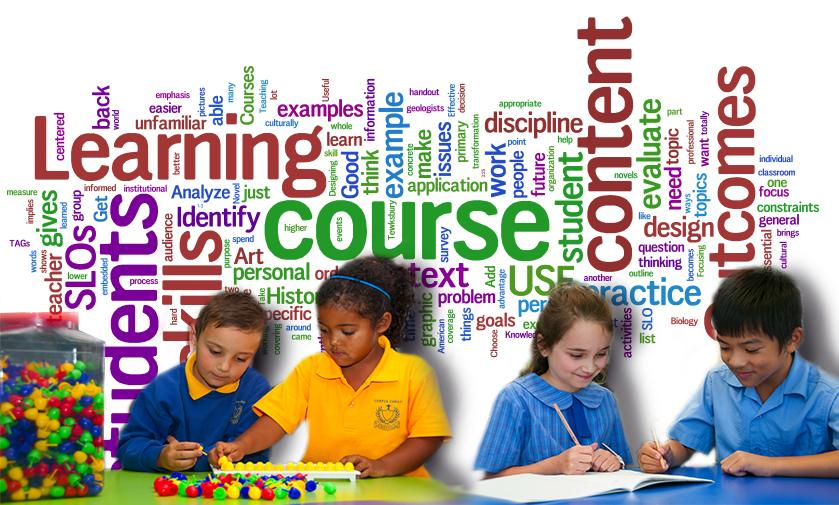# Learning and Teaching

## Mathematics

The proficiencies of Understanding, Fluency, Problem Solving and Reasoning are fundamental to learning Mathematics and working mathematically and are applied across all three strands Number and Algebra, Measurement and Geometry, and Statistics and Probability. When we teach Maths, we aim to assist students to develop these proficiencies through using different types of practices such as Number Talks, games, and worded problems.

Problem-solving is the ability of students to make choices, interpret, formulate, model and investigate problem situations, select and use technological functions and communicate solutions effectively. Students pose and solve problems when they:

• use Mathematics to represent unfamiliar or meaningful situations
• apply their existing strategies to seek solutions
• verify that their answers are reasonable.

In some Learning Communities, your child will be taught the process of RUCSAC to solve problems.

There are also a number of strategies to choose from when problem-solving.